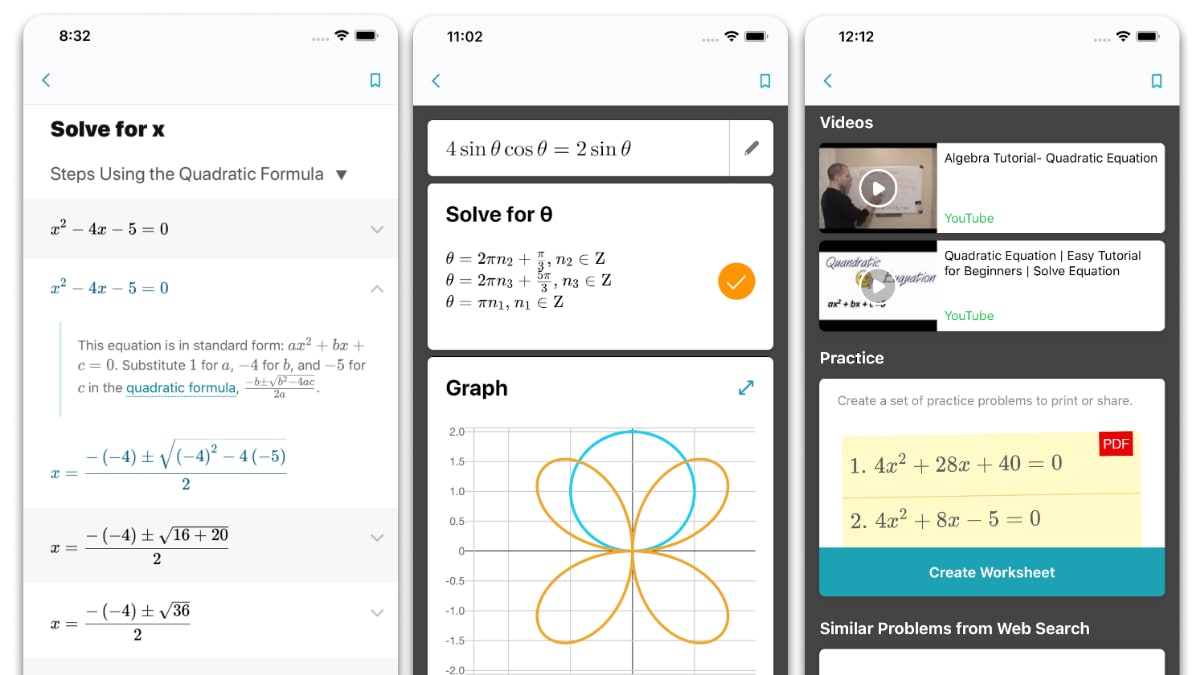# Microsoft Math Solver App Launched, Uses AI to Help Solve Mathematical Problems Instantly

Microsoft Math Solver app has been released as an app-in-one solution to solve mathematical problems on Android or iOS devices. The app uses artificial intelligence (AI) to recognise math problems and provides solutions for a wide range of concepts, ranging from elementary arithmetic and quadratic equations to calculus and statistics. Users can add their problems to the Microsoft Math Solver app directly by drawing, scanning, or typing. The app is also designed to provide graphs and step-by-step explanation.

To begin with the Microsoft Math Solver app, all you need to do is either capture a photo including your math problem or type your problem on the screen by using the built-in scientific calculator. You can also add your problem statements by drawing on the display of your smartphone or tablet using your finger or a stylus. The app will then use its AI algorithms to start recognising the text and then show the problem on the screen.

Once it recognises and shows the problem, the Microsoft Math Solver app provides you with instant solutions and highlights step-by-step guidance to let you learn how to solve your problems even without the app.

Microsoft has provided support for 22 languages including 12 Indian languages such as Assamese, Bengali, Gujarati, Hindi, Kannada, Marathi, Punjabi, and Tamil among others. There are also international languages such as German, Spanish, and Russian. The wide language support would be helpful to let anyone understand how various math concepts work.

Further, the Microsoft Math Solver app provides additional learning resources, including video tutorials and similar worksheets. These make it easier for students to learn and practice on distinct problems.Microsoft Math Solver provides various ways to easily solve mathematical problems

Microsoft has also included interactive graphs to help you understand correlations easily. Similarly, you can scan and plot X-Y data tables for linear and non-linear functions.

The Microsoft Math Solver app also lists similar problems from Web search using Bing. Further, you’ll get related concepts on the basis of your problems. You can also bookmark problems or view history. Furthermore, the app lists some examples to let you practice concepts such as algebra, fractions, rational equations, radical equations, arithmetic, trigonometry, matrix multiplication, and differentiation among various others.

In our brief usage with the Microsoft Math Solver app, we found that it certainly comes as a handy option to solve math problems for students. The way it recognises problems through an image, handwriting, and text makes it a distinct offering.

### Supported problems on Microsoft Math Solver app:

• Elementary: arithmetic, real, complex numbers, LCM, GCD, factors, roman numerals
• Pre-Algebra: radicals and exponents, fractions, matrices, determinants
• Algebra: quadratic equations, system of equations, inequalities, rational expressions, linear, quadratic and exponential graphs
• Word problems on math concepts, number theory, probability, volume, surface area
• Basic Calculus: Summations, Limits, derivatives, integrals
• Statistics: Mean, Median, Mode, Standard Deviation, permutations, combinations

You can try solving your math problems on the Microsoft Math Solver app by downloading its package from Apple App Store or Google Play. The app is compatible with devices running at least Android 5.0 Lollipop or iOS 11.0.